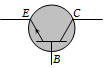Least doped region in a transistor

(a) Either emitter or collector

(b) Base

(c) Emitter

(d) Collector

Concept Questions :-

Transistor
High Yielding Test Series + Question Bank - NEET 2020

Difficulty Level:

The transistors provide good power amplification when they are used in

(a) Common collector configuration

(b) Common emitter configuration

(c) Common base configuration

(d) None of these

Concept Questions :-

Applications of Transistor
High Yielding Test Series + Question Bank - NEET 2020

Difficulty Level:

The transfer ratio of a transistor is 50. The input resistance of the transistor when used in the common-emitter configuration is 1 K$\mathrm{\Omega }$. The peak value for an A.C input voltage of 0.01 V peak is
(a) 100 $\mathrm{\mu }$A                        (b) 0.01 mA
(c) 0.25 mA                       (d) 500 $\mathrm{\mu }$A

Concept Questions :-

Transistor
High Yielding Test Series + Question Bank - NEET 2020

Difficulty Level:

For a transistor the parameter $\mathrm{\beta }$ = 99. The value of the parameter $\mathrm{\alpha }$ is

(a) 0.9                 (b) 0.99
(c) 1                    (d) 9

Concept Questions :-

Transistor
High Yielding Test Series + Question Bank - NEET 2020

Difficulty Level:

In a PNP transistor the base is the N-region. Its width relative to the P-region is

(a) Smaller           (b) Larger
(c) Same              (d) Not related

Concept Questions :-

Transistor
High Yielding Test Series + Question Bank - NEET 2020

Difficulty Level:

A common emitter amplifier is designed with NPN transistor ($\mathrm{\alpha }$ = 0.99). The input impedance is 1 K$\mathrm{\Omega }$ and load is 10 K$\mathrm{\Omega }$. The voltage gain will be

(a) 9.9                           (b) 99
(c) 990                          (d) 9900

Concept Questions :-

Applications of Transistor
High Yielding Test Series + Question Bank - NEET 2020

Difficulty Level:

The symbol given in figure represents(a) NPN transistor
(b) PNP transistor
(c) Forward biased PN junction diode
(d) Reverse biased NP junction diode

Concept Questions :-

Transistor
High Yielding Test Series + Question Bank - NEET 2020

Difficulty Level:

The most commonly used material for making transistor is

(a) Copper                 (b) Silicon
(c) Ebonite                 (d) Silver

Concept Questions :-

Transistor
High Yielding Test Series + Question Bank - NEET 2020

Difficulty Level:

An NPN-transistor circuit is arranged as shown in figure. It is(a) A common base amplifier circuit
(b) A common emitter amplifier circuit
(c) A common collector amplifier circuit
(d) Neither of the above

Concept Questions :-

Transistor
High Yielding Test Series + Question Bank - NEET 2020

Difficulty Level:

The part of a transistor which is heavily doped to produce a large number of majority carriers is-

1. Base

2. Emitter

3. Collector

4. None of these

Concept Questions :-

Transistor